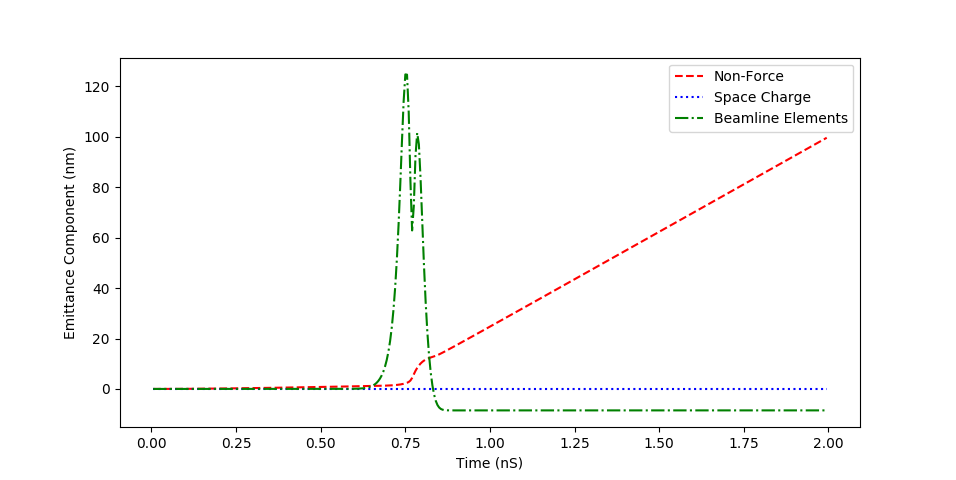Tags

# Solenoid, Chromatic Aberration

## Description

This test case represents a beam with energy spread passing through a solenoid. The emittance is observed to increase due to chromatic aberration.

## GPT Input

```# This GPT input file describes a beam with energy spread that is focused by a
# solenoid.  Its purpose is to investigate the effect of chromatic aberration
# on the emittance components.

# Define the beam parameters
gamma = 2;
gamma_relative_width = 0.005;
length = 1e-6;
total_charge = -10E-15;
number_of_particles = 1000;
initial_emittance = 1E-9;

# Define element parameters
solenoid_current = 5000;

# Start the beam
setparticles("beam", number_of_particles, me, qe, total_charge);
setphidist("beam", "u", 0, 2*pi);
setGdist("beam", "g", gamma, gamma*gamma_relative_width, 20, 20);
setzdist( "beam", "g", 0, length, 3, 3) ;

# Set its initial emittance to 1nm
setGBxdist("beam", "g", 0.0, 1e-3, 3.0, 3.0);
setGBxemittance("beam", initial_emittance);
setGBydist("beam", "g", 0.0, 1e-3, 3.0, 3.0);
setGByemittance("beam", initial_emittance);

# Insert a solenoid
solenoid("wcs", "z", 0.2, 0.01, solenoid_current);

# Compute the emittance components
emittance_component("4d space charge", "false");
emittance_component("4d beamline element", "false");
emittance_component("4d non-force", "false");

# Set the solver tolerance
dtmax = 2.5e-14;

# Print touts
tout(0.0, 2.0e-9, 5e-12);```

## Plots

### Emittance ComponentsTopic revision: r1 - 13 Aug 2018, ChristopherPierce
Copyright © by the contributing authors. All material on this collaboration platform is the property of the contributing authors.
Ideas, requests, problems regarding CLASSE Wiki? Send feedback